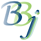# Substrings

A string variable can be subscripted to reference any part of the string. A substring has a starting position and a length. These subscripts are enclosed in parentheses.

You can use one or two subscripts to specify a substring. The first subscript is one-based and determines the first character position of the substring. The second subscript determines the length of the substring. If you do not specify a second subscript, PRO/5 assumes that the length of the substring is the remainder of the string.

The following rules apply to substrings:

• The first subscript cannot be negative.

• The second subscript cannot be negative in PRO/5. In BBj, a negative second subscript is treated as if no second subscript was specified at all.

• The length of a substring can be zero.

• The referenced substring must exist.

• If a string is N bytes long, the first subscript can be N+1 only if the length given is zero.

• If both subscripts are present and both are zero, PRO/5 returns a null string. For example, A\$(0,0) is null. This is the only case in which the first subscript can be zero.

Some examples of substrings:

A\$(1,5)
NAME\$(10)
XYZ\$[3,4](1,5)

In the last example notice the combination of array subscripts and substring specification.## BBj-Specific Information

By default, BBj considers X\$(pos,-1) to be equivalent to X\$(pos), returning the substring from pos through the end of the string. In BBj 14 and higher, the LEGACY_SUBSTRING_LENGTH !COMPAT setting can be set to TRUE to make substring references with a negative length throw!ERROR=47for compatibility with PRO/5.

In BBj 15 and higher, setting the THOROUGHBRED_IF47 !COMPATsetting to TRUE enables IF47 emulation for Thoroughbred Basic conversions. In this emulation mode, substring references in an IF condition that would normally generate !ERROR=47 are instead evaluated to "". For example:

compat\$ = stbl("!COMPAT","THOROUGHBRED_IF47=TRUE")

let x\$ = ""

if x\$(10,10) = "" then print "if47"

if len(cvs(x\$(10,10),3)) = 0 then print "if47"